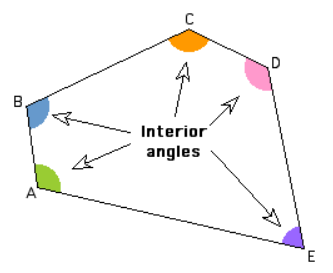# What do interior angles of a polygon mean?

An Interior Angle is an angle inside a shape. The interior angles of a polygon are those angles at each vertex that are on the inside of the polygon. For a regular polygon, by definition, all the interior angles are the same.To find the sum of interior angles of a polygon, multiply the number of triangles in the polygon by 180°. The formula for calculating the sum of interior angles is $( n − 2 ) × 180°$  where n is the number of sides. All the interior angles in a regular polygon are equal.

The sum of exterior angles of a polygon is 360°.

Updated on: 10-Oct-2022

36 Views